# Split full name to first and last name

This post will guide you how to split the full name to the first name and last name in the different cells in excel. How to create an excel formula to split the full name to first name and last name. How to get the middle name from the full name string using a formula.

## Split full name to first and last name

Assuming that you have a full name list in a table or range B2:B3 and you want to get the first name from this full name list and then put it in another cell. Or you just only want to extract the last name from the full name string, and put it in other cell. You can use a combination of the LEFT function and FIND function to create a formula to extract the first name from the full name.

You can create another formula based on the RIGHT function, the LEN function, the FIND function and the SUBSTITUTE function to extract the last name.

### Split full name to get first name

You can write down the following formula to get the first name:

`=LEFT(B2, FIND(" ",B2,1)-1)`

Let’s see how this formula works:

= FIND(” “,B2,1)-1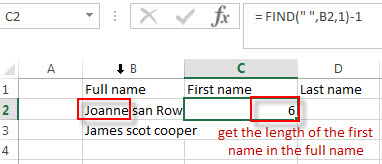This formula returns the position of the first space character in the full name string. And the number is subtracted 1 to get the length of the first name in the full name string.

=LEFT(B2, FIND(” “,B2,1)-1)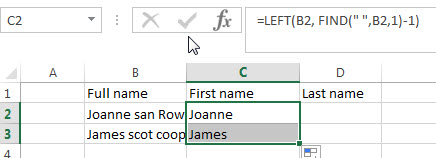This formula will extract the first name from the full name string based on the length of the first name returned by the FIND function.

### Split full name to get Last name

You can use the following formula to extract the last name:

`=RIGHT(B2, LEN(B2)- FIND("#",SUBSTITUTE(B2," ","#",2),1))`

Let’s see how this formula works:

= SUBSTITUTE(B2,” “,”#”,2)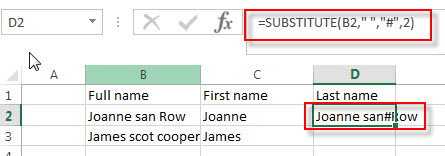This formula locate the position of the second space string and then replace it with hash character. It goes into the FIND function as its Text argument.

= FIND(“#”,SUBSTITUTE(B2,” “,”#”,2),1)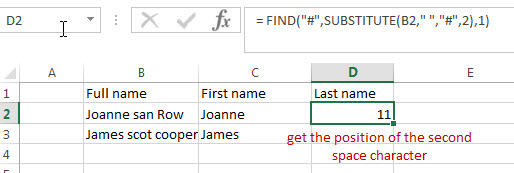This formula returns the position of the hash character in the text string that returned by the SUBSTITUTE function. The number is also the position of the second space character in the full name string.

= LEN(B2)- FIND(“#”,SUBSTITUTE(B2,” “,”#”,2),1)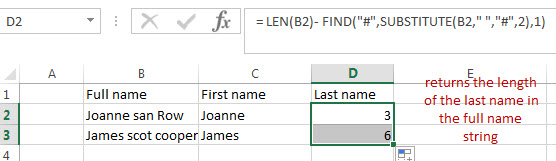This formula returns the length of the last name in the full name string.

=RIGTH()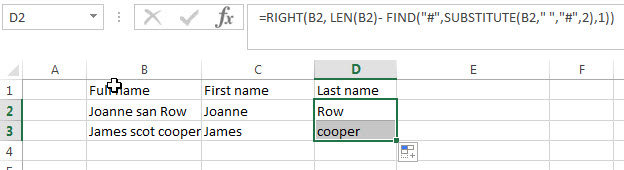This formula extracts the right most characters based on the length of the last name.

### Split full name to get Middle name

If you want to extract the middle name from the full name string, you can write down the following formula:

`= MID(B2,FIND(" ",B2)+1,FIND("#",SUBSTITUTE(B2," ","#",2),1)- FIND(" ",B2,1)-1)`

Let’s see how this formula works:This formula returns the position of the hash character in the text string that returned by the SUBSTITUTE function. The number is also the position of the second space character in the full name string.

= FIND(” “,B2,1)-1This formula returns the position of the first space character in the full name string. And the number is subtracted 1 to get the length of the first name in the full name string.

=FIND(“#”,SUBSTITUTE(B2,” “,”#”,2),1)- FIND(” “,B2,1)-1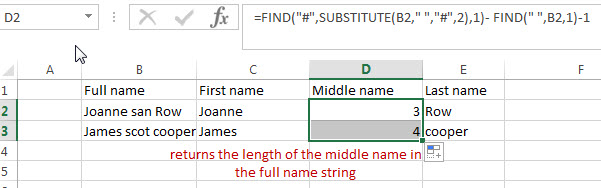This formula returns the length of the middle name in the full name string.  And it goes into the MID function as its num_chars argument.

= FIND(” “,B2)+1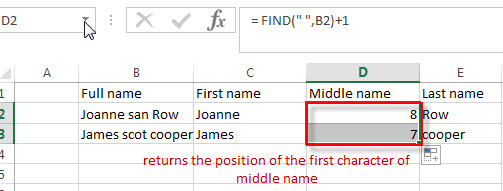This formula returns the position of the first character of middle name in the full name string. It goes into the MID function as its start_num argument.

=MID()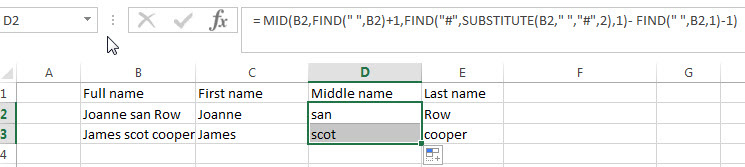This formula extracts the middle name from the full name based on the start_num and num_chars arguments.

### Related Formulas

• Get first word from text string
If you want to extract the first word from a text string in a cell, you can use a combination of the IF function, the ISERR function, the LEFT function and the FIND function to create a complex excel formula..…
• Split Multiple Lines from a Cell into Rows
If you have multiple lines in a cell and each line is separated by line break character or press “alt + enter” key while you entering the text string into cells, and you need to extract the multiple lines into the separated rows or columns, you can use a combination with the TRIM function, the MID function, the SUBSTITUTE function, the REPT function, the LEN function to create a complex excel formula..…
• Get last word from text string
If you want to get the last word from a text string, you can create an excel formula based on the RIGHT function, the LEN function, the FIND function and the SUBSTITUTE function..…
• Extract nth word from text string
If you want to extract the nth word from a text string in a single cell, you can create an excel formula based on the TRIM function, the MID function, the SUBSTITUTE function, the REPT function and the LEN function..…
• count specific words in a cell or a range
If you want to count the number of a specific word in a single cell, you need to use the SUBSTITUTE function to remove all that certain word in text string, then using LEN function to calculate the length of the substring that without that specific word.…
• Extract word that starting with a specific character
Assuming that you have a text string that contains email address in Cell B1, and if you want to extract word that begins with a specific character “@” sign, you can use a combination with the TRIM function, the LEFT function, the SUBSTITUTE function, the MID function, the FIND function, the LEN function and the REPT function to create an excel formula.…

### Related Functions

• Excel LEFT function
The Excel LEFT function returns a substring (a specified number of the characters) from a text string, starting from the leftmost character.The LEFT function is a build-in function in Microsoft Excel and it is categorized as a Text Function.The syntax of the LEFT function is as below:= LEFT(text,[num_chars])…t)…
• Excel FIND function
The Excel FIND function returns the position of the first text string (sub string) within another text string.The syntax of the FIND function is as below:= FIND(find_text, within_text,[start_num])…
• Excel RIGHT function
The Excel RIGHT function returns a substring (a specified number of the characters) from a text string, starting from the rightmost character.The syntax of the RIGHT function is as below:= RIGHT (text,[num_chars])…
• Excel Substitute function
The Excel SUBSTITUTE function replaces a new text string for an old text string in a text string.The syntax of the SUBSTITUTE function is as below:= SUBSTITUTE  (text, old_text, new_text,[instance_num])….
• Excel LEN function
The Excel LEN function returns the length of a text string (the number of characters in a text string).The syntax of the LEN function is as below:= LEN(text)…
• Excel MID function
The Excel MID function returns a substring from a text string at the position that you specify.The syntax of the MID function is as below:= MID (text, start_num, num_chars)….
Related Posts

Find and Replace Multiple Values

This post will guide you how to find and replace multiple values at once with VBA macro or using formula  in Excel. How do I make multiple find and replace in Excel. Suppose that you have a few cells containing ...

Extract Last Two Words From Multiple Cells

Just assume that you have a few cells containing values/words and you want to extract the last two words from each cell into another separate cell; then you might think that it's not a big deal; because you would prefer ...

Extract Multiple Lines From A Cell

Suppose that you have listed some text in a single cell which is separated by the line break(you can do it by pressing ALT + ENTER after entering the text), and now you want to extract multiple lines of text ...

How to Remove All Asterisk Characters from Cells in Excel

Sometimes when we opening an excel spreadsheet to check data, we may notice that there are some asterisks exist in texts for security or other reasons. If you feel these special characters are invalid you can remove them from cells. ...

How to Convert Date Format from Dot MM.DD.YYYY to Slash MM/DD/YYYY in Excel

We often enter dates in excel tables, and we can enter current date by quickly press ‘Ctrl+;’ simultaneously. If in some cases we enter a date format that is not what we want, we have to switch the date format ...

Sidebar